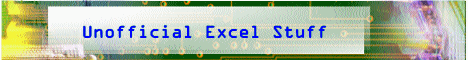Book

Suggestions

Delivered by FeedBurner

Bloglines

1906
2006

OfficeZealot

Scobleizer

TechRepublic

SpyJournalWeb http://www.klippert.comSunday, June 14, 2009 – Permalink –

## Julian Dates

### Gregorian to/from JulianJulian dates refer to the number of days from the first of the year and the number of days until the end of the year.

The year -45 has been called the "year of confusion," because in that year Julius Caesar inserted 90 days to bring the months of the Roman calendar back to their traditional place with respect to the seasons. This was Caesar's first step in replacing a calendar that had gone badly awry. Caesar created a solar calendar with twelve months of fixed lengths and a provision for an intercalary day to be added every fourth year. As a result, the average length of the Julian calendar year was 365.25 days.

The Gregorian (Pope Gregory XIII) calendar is based on a cycle of 400 years, which comprises 146,097 days. Since 146,097 is evenly divisible by 7. Dividing 146,097 by 400 yields an average length of 365.2425 days per calendar year, which is a close approximation to the length of the tropical year. The Gregorian calendar accumulates an error of one day in about 2500 years.

Calendars by L. E. Doggett

From Chip Pearson's site CPearson.com:

"Many applications (especially mainframe systems) store dates in the Julian format, which is a 5-digit number, consisting of a 2-digit year and a 3-digit day-of-year number. For example, 24-August-1999 is stored as 99236, since 24-August is the 236th day of the year. Excel does not support Julian dates directly, but you can use them with only a few fairly simple formulas.

### Converting A Standard Date To A Julian Date

The formula below will convert a standard Excel date in A1 to a Julian Date.

`=RIGHT(YEAR(A1),2)&TEXT(A1-DATE(YEAR(A1),1,0),"000")`

This formula takes the 2 right-most characters of the YEAR of the date in A1, and then appends the number of days between the date in A1 and the 0th day of that year. The TEXT function formats the day-of-year number as three digits, with leading zeros if necessary.

### Converting A Julian Date To A Standard Date

The formula below will convert a Julian date to a standard Excel date.

`=DATE(IF(0+(LEFT(A1,2))<30,2000,1900)+LEFT(A1,2),1,RIGHT(A1,3))`

If the year digits of the Julian date are less than 30 (i.e., 00 to 29), the date is assumed to be a 2000 century year. If the year digits of the Julian date are greater than or equal to 30 (i.e., 30 to 99), the date is assumed to be a 1900 century year. This formula works by taking advantage of the fact that the DATE function can handle days beyond the "normal" days in a month. For example, DATE correctly computes 100-Jan-1999 to be 10-April-1999.

These Julian dates must have the leading zero or zeros for years between 2000 and 2009. For example the 123rd day of 2000 must be entered as 00123. Format the cell as TEXT before entering the data, or enter an apostrophe before the Julian date -- e.g., '00123. This will prevent Excel from treating the Julian date as a number and suppressing the leading zeros."

US Naval Observatory has this definition (and a calculator):

Julian dates (abbreviated JD) are simply a continuous count of days and fractions since noon Universal Time on January 1, 4713 BCE (on the Julian calendar). Almost 2.5 million days have transpired since this date.

April 29, 2004 at 6:00 AM would be 2453854.75

See all Topics

Labels: ,

<Doug Klippert@ 3:43 AM